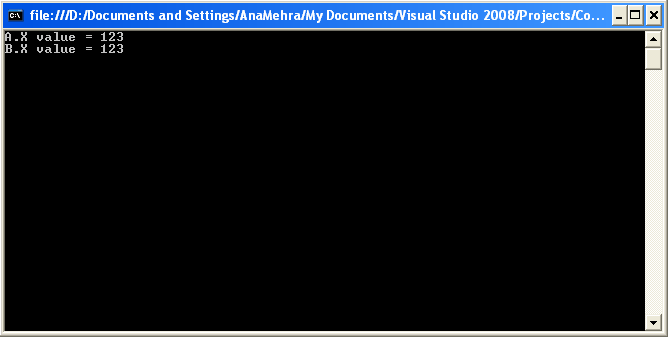# Constants in C#

A constant is a variable modifier that represents a constant value, a value that can be computed at compile-time. A constant declaration introduces one or more constants of a given type.

A constant declaration can declare multiple constants (as in Listing 5.18) in a process that is equivalent to multiple declarations of single constants with the same attributes, modifiers, and type.

Listing 5.18: Constants Example 1

class A
{
public const int X = 1, Y = 2, Z = 3;
}

class B
{
public const int X = 1;
public const int Y = 2;
public const int Z = 3;
}

Evaluating constant values in complex class hierarchies can be a bit confusing and warrants closer examination.

The declaration of constants can depend on other constants within the same program (see Listing 5.19) as long as the dependencies are not of a circular nature. The .NET framework automatically arranges constant declarations to evaluate the declarations in the appropriate order.

Listing 5.19: Constants Example 2

class A
{
public const int X = B.Z + 1;
public const int Y = 10;
}

class B
{
public const int Z = A.Y + 1;
}

In this example, the compiler first evaluates Y, then Z, and finally X, producing the values 10, 11, and 12. Constant declarations may depend on constants from other programs, but such dependencies are only possible in one direction. Referring to the example above, if A and B were declared in separate programs, it would be possible for A.X to depend on B.Z; however, B.Z could not then depend on A.Y.

A static read-only field is useful when a symbolic name for a constant value is desired—for example, when the type of the value is not permitted in a constant declaration or when the value cannot be computed at compile-time. Constants and read-only fields have different binary versioning semantics. When an expression references a constant, the value of the constant is obtained at compile-time. When an expression references a read-only field, the value of the field is not obtained until runtime.

Listings 5.20, 5.21, and 5.22 present a solid example of the difference between const and read-only in real-world applications.

Listing 5.20: ConstOut.cs , Constant Usage Example

using
System;

public
class A
{
public const int X = 123;
}

using
System;

public
class B
{
public static readonly int X = 123;
}

using
System;

public
class MyTest
{
public static void Main()
{
Console.WriteLine("A.X value = {0}", A.X);
Console.WriteLine("B.X value = {0}", B.X);
}
}

The code in these three examples produces the display shown in Figure 5.7.Figure 5.7: Screen Output Generated from Listings 5.20, 5.21, and 5.22

If you modify X in ConstOut.cs, then you have to compile both ConstOut.cs and ReadOnlyConst.cs consecutively. But if you modify X in ReadOnlyOut.cs, then you only need to compile ReadOnlyOut.cs; there is no need to recompile MyTest.cs. However, the execution speed of const is better than that of the static read-only.

Conclusion

Hope this article would have helped you in understanding constants. See other articles on the website on .NET and C#.The Complete Visual C# Programmer's Guide covers most of the major components that make up C# and the .net environment. The book is geared toward the intermediate programmer, but contains enough material to satisfy the advanced developer.